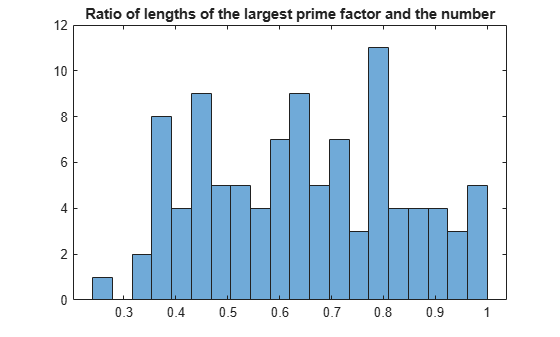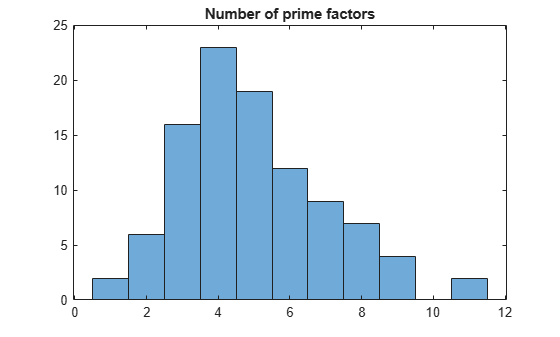# Prime Factorizations

This example shows how to use some elementary functions on `sym` objects using the Symbolic Math Toolbox™.

The built-in integer types of MATLAB® are suitable for integers smaller than 2^64. However, we want to carry out statistical investigations on prime factorizations of larger integers. To do this, we use symbolic integers because their size is unlimited. Investigate the integers between ${N}_{0}+1$ and ${N}_{0}+100$, where ${N}_{0}=3*1{0}^{23}$. The built-in data types cannot store such values exactly. Thus, wrap the innermost number with `sym` to use symbolic representation in the calculations. This avoids rounding or overflow errors:

```N0 = 3*sym(10)^23; disp(['Roundng error for doubles: ' char(3*10^23 - N0)]);```
```Roundng error for doubles: -25165824 ```
`disp(['Overflow error for integers: ' char(3*uint64(10)^23 - N0)]);`
```Overflow error for integers: -299981553255926290448385 ```

In arithmetical operations, symbolic numbers can be combined with doubles, and the conversion takes place before the operation. Thus, the following definition cannot cause rounding errors:

`A = N0 + (1:100);`

Compute the prime factorizations of the elements of `A` using `factor`. The number of prime factors differs. Arrays cannot contain vectors of different lengths, but cell arrays can. To avoid memory re-allocations, initialize the cell array first, then compute the factorizations in a loop:

```Bcell = cell(1, 100); for i=1:100 Bcell{i} = factor(A(i)); end```

A more efficient approach is to use `arrayfun`. Setting `UniformOutput` to `false` returns the result as a cell array.

`Bcell = arrayfun(@factor, A, 'UniformOutput', false);`

For example, the first prime factorizations are:

`Bcell{1:5}`
`ans = $\left(\begin{array}{cccc}13& 43& 233& 2303316007278478583\end{array}\right)$`
`ans = $\left(\begin{array}{ccccccc}2& 17& 173& 991& 1223& 244939& 171805943\end{array}\right)$`
`ans = $\left(\begin{array}{cccccc}3& 11& 47& 139& 2531& 549797184491917\end{array}\right)$`
`ans = $\left(\begin{array}{ccccc}2& 2& 330131& 2953837& 76910994983\end{array}\right)$`
`ans = $\left(\begin{array}{cccc}5& 6271& 2650266823& 3610146697\end{array}\right)$`

Obtain the largest prime factor using `max`. Note that if the output consists of sym objects, the option `UniformOutput` always has to be set to `false` even if the output is uniform.

`Mcell = cellfun(@max, Bcell, 'UniformOutput', false);`

For example, the first maximal prime factors are:

`Mcell{1:5}`
`ans = $2303316007278478583$`
`ans = $171805943$`
`ans = $549797184491917$`
`ans = $76910994983$`
`ans = $3610146697$`

Convert the cell array to a symbolic vector, and investigate the ratio of the lengths of the largest prime factor and the number as a whole. You can apply arithmetical operations elementwise to symbolic vectors, in the same way as for doubles. Note that most statistical functions require their arguments to be double-precision numbers.

```M = [Mcell{:}]; histogram(double(log(M)./log(A)), 20); title('Ratio of lengths of the largest prime factor and the number');```In the same way, now investigate the distribution of the number of prime factors. Here, the result contains uniform numeric data. Therefore, you do not need to set `UniformOutput` to `false`.

```omega = cellfun(@numel, Bcell); histogram(omega); title('Number of prime factors');```The interval under investigation contains two prime numbers:

`A(omega == 1)`
`ans = $\left(\begin{array}{cc}300000000000000000000037& 300000000000000000000049\end{array}\right)$`

We check that the maximal prime factors are about equally often in the residue classes 1 and 3 modulo 4. Note that equations of sym objects are symbolic objects themselves and not logical values; we have to convert them before we can sum them:

`sum(logical(mod(M, 4) == 1))`
```ans = 49 ```
`sum(logical(mod(M, 4) == 3))`
```ans = 51 ```